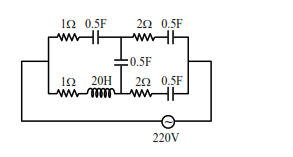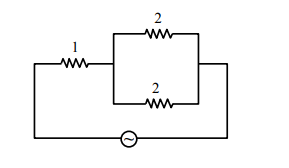# At very high frequencies, the effective impendanceQuestion:

At very high frequencies, the effective impendance

of the given circuit will be $\Omega .$Solution:

$\mathrm{X}_{\mathrm{L}}=2 \pi \mathrm{fL}$

$\mathrm{f}$ is very large

$\therefore \mathrm{X}_{\mathrm{L}}$ is very large hence open circuit.

$X_{C}=\frac{1}{2 \pi f C}$

$\mathrm{f}$ is very large.

$\therefore \mathrm{X}_{\mathrm{C}}$ is very small, hence short circuit.

Final circuit$Z_{\mathrm{cq}}=1+\frac{2 \times 2}{2+2}=2$